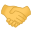# SolvedWhat does ^@ means here?! (flex problem)

#### hruodr

##### Well-Known Member

Reaction score: 47
Messages: 380

For deleting too much blanks and \n on a null terminated string saved in a char array yytext I am using:

Code:
``````int trim() {int r=0; int w=0; int b=0; char c;
while ((c=yytext[w++]=yytext[r++])!=0) {
if (c=='\n') c=yytext[w-1]=' ';
if (c==' ') ++b; else b=0;
if (b>1) --w;}
return 0;}``````
The position of the null moves to the left, what remains to the right should play no role. I thought!

yylex variable in lex should be an array of chars, as far as I knew!

I applied the following lex script to a specific input:

Code:
``````%array
%{
#include <stdio.h>
int trim() {int r=0; int w=0; int b=0; char c;
while ((c=yytext[w++]=yytext[r++])!=0) {
if (c=='\n') c=yytext[w-1]=' ';
if (c==' ') ++b; else b=0;
if (b>1) --w;}
return 0;}
int yywrap() {return 1;}
%}
%%
...[ \n]*"<orth type=\"arrow\">" {trim(); ECHO; putchar('\n');}
.|\n                             ;
%%
int main() {yylex();}``````
trim deleted 8 matches of [ \n]* and returned:

↓ <orth type="arrow">^@rrow">

trim() deleted 7 blanks and 1 \n, copied the rest to the left, including the terminating 0 as expected, but the strange thing is that the new 0 does not terminate the string, but the original one.

Why?!

The problem is solved substituting `ECHO; putchar('\n');` with `printf("%s\n",yytext);`.

But is there an explanation?

OP
OP
H

#### hruodr

##### Well-Known Member

Reaction score: 47
Messages: 380

I got it: ECHO outputs yyleng bytes of yytext.Remark: this is ` flex` in FreeBSD, not the original ` lex`: according to /usr/share/doc/psd/16.lex ECHO in ` lex` is equivalent to printf("%s", yytext);

•SirDice